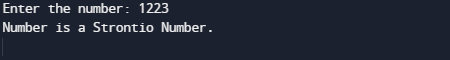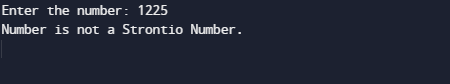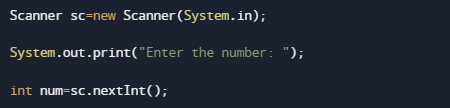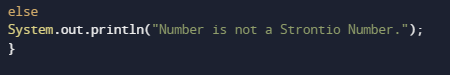# Program to check Strontio Number in Java

In this tutorial you will learn about the Program to check Strontio Number in Java and its application with practical example.

In this tutorial, we will learn to create a Java Program to check Strontio Number in Java using Java programming.

## Prerequisites

Before starting with this tutorial we assume that you are best aware of the following Java programming topics:

• Java Operators.
• Basic Input and Output function in Java.
• Class and Object in Java.
• Basic Java programming.
• If-else statements in Java.
• For loop in Java.
• User define functions.

## What is Strontio numbers?

Strontio numbers is a four digits numbers which is whenever we multiply Strontio number with 2 it always gives the same digit at the hundreds and tens place.But the inputted number must be a four-digit number.

Example:
1221 = >  1221*2  => 2442 is a Strontio number.

1224 =>  1234*2  => 2468 Not a Strontio Number.

## Program to check Strontio Number in Java

In this program we will check given number is Strontio Number or not. We would first declared and initialized the required variables. Next, we would prompt user to input the value. Later we will find Strontio Number.

Strontio Number

##Not a Strontio NumberIn the above program, we have first declared and initialized a set variables required in the program.

• num = it will hold entered number.

After that take a value of number from user and store that number into variable “num”.as shown in image below.Now, the logic behind to be a strontio number is when we multiplied number by 2 it  gives same number in Tens and Hundreds place.Let have a look at the example

Example: 1223, 1223 × 2 = 2446, So  4 and 4 are in Ten’s and Hundreds Place the number is a Strontio Number.After Comparing both remainder and quotient. If they are equal, the given number (num) is strontio, else is not a Strontio Number.So we have seen in our program that  value at tens and  value at  hundreds place have the same digits. Hence, the given number 1223 is a strontio number. Similarly, we can check other numbers also that they are Strontio or not.

In this tutorial we have learn about the Program to check Strontio Number in Java and its application with practical example. I hope you will like this tutorial.